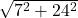## The hypotenuse of a right angle triangle is 50cm and the legs are bin the ratio of 7:24. Find the area of right angle triangle.

Question

The hypotenuse of a right angle triangle is 50cm and the legs are bin the ratio of 7:24. Find the area of right angle triangle.

in progress 0
2 months 2021-07-22T12:41:48+00:00 1 Answers 5 views 0

84 cm^2

Step-by-step explanation:

area of right triangle formula= 1/2 (base*height)

In order to find the area, you need the base and the height

The question included that the legs ratio are 7 to 24.

If you do, you get 25 (Pythagorean theorem)

so the base and heights are 7 and 24

1/2 (7*24)= 84